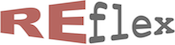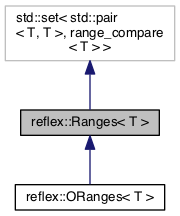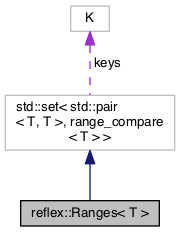reflex::Ranges< T > Class Template Reference updated Mon Jan 23 2023 by Robert van Engelen
reflex::Ranges< T > Class Template Reference

RE/flex Ranges template class. More...

`#include <ranges.h>`

Inheritance diagram for reflex::Ranges< T >:[legend]
Collaboration diagram for reflex::Ranges< T >:[legend]

## Public Types

typedef T bound_type
Type of the bounds. More...

typedef std::set< std::pair< T, T >, range_compare< T > > container_type
Synonym type defining the base class container std::set. More...

typedef container_type::value_type value_type
Synonym type defining the base class container std::set::value_type. More...

typedef container_type::key_compare key_compare
Synonym type defining the key/value comparison std::set::key_compare. More...

typedef container_type::value_compare value_compare

typedef container_type::iterator iterator
Synonym type defining the base class container std::set::iterator. More...

typedef container_type::const_iterator const_iterator
Synonym type defining the base class container std::set::iterator. More...

## Public Member Functions

Ranges ()
Construct an empty range. More...

Ranges (const value_type &r)
Construct a copy of a range [lo,hi]. More...

Ranges (const bound_type &lo, const bound_type &hi)
Construct a range [lo,hi]. More...

Ranges (const bound_type &val)
Construct a singleton range [val,val]. More...

std::pair< iterator, bool > insert (const value_type &r)
Update ranges to include range [lo,hi] by merging overlapping ranges into one range. More...

std::pair< iterator, bool > insert (const bound_type &lo, const bound_type &hi)
Update ranges to include range [lo,hi] by merging overlapping ranges into one range. More...

std::pair< iterator, bool > insert (const bound_type &val)
Update ranges to include the range [val,val]. More...

const_iterator find (const bound_type &lo, const bound_type &hi) const
Find the first range [lo',hi'] that overlaps the given range [lo,hi], i.e. lo <= hi' and lo' <= hi. More...

const_iterator find (const bound_type &val) const
Find the range [lo',hi'] that includes the given value val, i.e. lo' <= val <= hi'. More...

Rangesoperator|= (const Ranges &rs)
Update ranges to insert the given range set, where this method has lower complexity than iterating insert() for each range in rs. More...

Rangesoperator+= (const Ranges &rs)
Update ranges to insert the ranges of the given range set, same as Ranges::operator|=(rs). More...

Rangesoperator&= (const Ranges &rs)
Update ranges to intersect the ranges with the given range set. More...

Ranges operator| (const Ranges &rs) const
Returns the union of two range sets. More...

Ranges operator+ (const Ranges &rs) const
Returns the union of two range sets, same as Ranges::operator|(rs). More...

Ranges operator& (const Ranges &rs) const
Returns the intersection of two range sets. More...

bool operator< (const Ranges &rs) const
True if this range set is lexicographically less than range set rs. More...

bool operator> (const Ranges &rs) const
True if this range set is lexicographically greater than range set rs. More...

bool operator<= (const Ranges &rs) const
True if this range set is lexicographically less or equal to range set rs. More...

bool operator>= (const Ranges &rs) const
True if this range set is lexicographically greater or equal to range set rs. More...

bool any () const
Return true if this set of ranges contains at least one range, i.e. is not empty. More...

bool intersects (const Ranges &rs) const
Return true if this set of ranges intersects with ranges rs, i.e. this set has at least one range [lo',hi'] that overlaps with a range [lo,hi] in rs such that lo <= hi' and lo' <= hi. More...

bool contains (const Ranges &rs) const
Return true if this set of ranges contains all ranges in rs, i.e. rs is a subset of this set which means that for each range [lo,hi] in rs, there is a range [lo',hi'] such that lo' <= lo and hi <= hi'. More...

bound_type lo () const
Return the lowest value in the set of ranges (the set cannot be empty) More...

bound_type hi () const
Return the highest value in the set of ranges (the set cannot be empty) More...

## Detailed Description

### template<typename T> class reflex::Ranges< T >

RE/flex Ranges template class.

The `std::set` container is the base class of this Ranges class. Value ranges [lo,hi] are stored in the underlying `std::set` container as a pair of bounds `std::pair(lo, hi)`.

Ranges in the set are mutually disjoint (i.e. non-overlapping). This property is maintained by the `reflex::Ranges` methods.

The `Ranges::value_type` is `std::pair<bound_type,bound_type>` with `Ranges::bound_type` the template parameter type `T`.

The `reflexx::Ranges` class introduces several new methods in addition to the inherited `std::set` methods:

• `std::pair<iterator,bool> insert(const bound_type& lo, const bound_type& hi)` updates ranges to include the range [lo,hi]. Returns an iterator to the range that contains [lo,hi] and a flag indicating that ranges was updated (true) or if the new range was subsumed by current ranges (false).
• `std::pair<iterator,bool> insert(const bound_type& val)` updates ranges to include the value [val,val]. Returns an iterator to the range that contains val and a flag indicating that ranges was updated (true) or if the new range was subsumed by current ranges (false).
• `const_iterator find(const bound_type& lo, const bound_type& hi) const` searches for the first range that overlaps with [lo,hi]. Returns an iterator to the range found or the end iterator.
• `const_iterator find(const bound_type& val) const` searches for the range that includes the given value. Returns an iterator to the range found or the end iterator.
• `Ranges& operator|=(const Ranges& rs)` inserts ranges rs. Returns reference to this object.
• `Ranges& operator+=(const Ranges& rs)` same as above.
• `Ranges& operator&=(const Ranges& rs)` update ranges to intersect with ranges rs. Returns reference to this object.
• `Ranges operator|(const Ranges& rs) const` returns union of ranges.
• `Ranges operator+(const Ranges& rs) const` same as above.
• `Ranges operator&(const Ranges& rs) const` returns intersection of ranges.
• `bool any() const` returns true if this set of ranges contains at least one range, i.e. is not empty.
• `bool intersects(const Ranges& rs) const` returns true if this set of ranges intersects ranges rs, i.e. has at least one range that overlaps with ranges rs.
• `bool contains(const Ranges& rs) const` returns true if this set of ranges contains all ranges rs, i.e. ranges rs is a subset.
• `bound_type lo()` returns the lowest value in the set of ranges.
• `bound_type hi()` returns the highest value in the set of ranges.
Warning
Using `std::set::insert()` instead of `Ranges::insert()` may result in overlapping ranges rather than merging ranges to produce disjoint non-overlapping ranges.

Example:

intervals.insert(1.0, 2.0); // insert 1.0..2.0
intervals.insert(2.0, 3.0); // insert 2.0..3.0
intervals.insert(-1.0, 0.0); // insert -1.0..0.0
std::cout << "Set of " << intervals.size() << " intervals:" << std::endl;
for (reflex::Ranges<float>::const_iterator i = intervals.begin(); i != intervals.end(); ++i)
std::cout << "[" << i->first << "," << i->second << "]" << std::endl;
if (intervals.find(2.5) != intervals.end())
std::cout << "2.5 is in intervals" << std::endl;
for (reflex::Ranges<float>::const_iterator i = intervals.find(0.0, 1.0); i != intervals.end() && i->first <= 1.0; ++i)
std::cout << "[" << i->first << "," << i->second << "] overlaps with [0.0,1.0]" << std::endl;
if (intervals.intersects(reflex::Ranges<float>(2.5, 10.0)))
std::cout << "intersects [2.5,10.0]" << std::endl;
if (intervals.contains(reflex::Ranges<float>(1.0, 2.5)))
std::cout << "contains [1.0,2.5]" << std::endl;

Output:

```Set of 2 intervals:
[-1,0]
[1,3]
2.5 is in intervals
[-1,0] overlaps with [0.0,1.0]
[1,3] overlaps with [0.0,1.0]
intersects [2.5,10.0]
contains [1.0,2.5]```

## Member Typedef Documentation

template<typename T>
 typedef T reflex::Ranges< T >::bound_type

Type of the bounds.

template<typename T>
 typedef container_type::const_iterator reflex::Ranges< T >::const_iterator

Synonym type defining the base class container std::set::iterator.

template<typename T>
 typedef std::set< std::pair,range_compare > reflex::Ranges< T >::container_type

Synonym type defining the base class container std::set.

template<typename T>
 typedef container_type::iterator reflex::Ranges< T >::iterator

Synonym type defining the base class container std::set::iterator.

template<typename T>
 typedef container_type::key_compare reflex::Ranges< T >::key_compare

Synonym type defining the key/value comparison std::set::key_compare.

template<typename T>
 typedef container_type::value_compare reflex::Ranges< T >::value_compare
template<typename T>
 typedef container_type::value_type reflex::Ranges< T >::value_type

Synonym type defining the base class container std::set::value_type.

## Constructor & Destructor Documentation

template<typename T>
 reflex::Ranges< T >::Ranges ( )
inline

Construct an empty range.

template<typename T>
 reflex::Ranges< T >::Ranges ( const value_type & r )
inline

Construct a copy of a range [lo,hi].

template<typename T>
 reflex::Ranges< T >::Ranges ( const bound_type & lo, const bound_type & hi )
inline

Construct a range [lo,hi].

Parameters
 lo lower bound hi upper bound
template<typename T>
 reflex::Ranges< T >::Ranges ( const bound_type & val )
inline

Construct a singleton range [val,val].

Parameters
 val value

## Member Function Documentation

template<typename T>
 bool reflex::Ranges< T >::any ( ) const
inline

Return true if this set of ranges contains at least one range, i.e. is not empty.

Returns
true if non empty, false if empty.
template<typename T>
 bool reflex::Ranges< T >::contains ( const Ranges< T > & rs ) const
inline

Return true if this set of ranges contains all ranges in rs, i.e. rs is a subset of this set which means that for each range [lo,hi] in rs, there is a range [lo',hi'] such that lo' <= lo and hi <= hi'.

Returns
true if this set contains rs.
Parameters
 rs ranges
template<typename T>
 const_iterator reflex::Ranges< T >::find ( const bound_type & lo, const bound_type & hi ) const
inline

Find the first range [lo',hi'] that overlaps the given range [lo,hi], i.e. lo <= hi' and lo' <= hi.

Returns
iterator to the first range that overlaps the given range, or the end iterator.
Parameters
 lo lower bound hi upper bound
template<typename T>
 const_iterator reflex::Ranges< T >::find ( const bound_type & val ) const
inline

Find the range [lo',hi'] that includes the given value val, i.e. lo' <= val <= hi'.

Returns
iterator to the range that includes the value, or the end iterator.
Parameters
 val value to search for
template<typename T>
 bound_type reflex::Ranges< T >::hi ( ) const
inline

Return the highest value in the set of ranges (the set cannot be empty)

Returns
highest value
template<typename T>
 std::pair reflex::Ranges< T >::insert ( const value_type & r )
inline

Update ranges to include range [lo,hi] by merging overlapping ranges into one range.

Returns
a pair of an iterator to the range and a flag indicating whether the range was inserted as new.
Parameters
 r range
template<typename T>
 std::pair reflex::Ranges< T >::insert ( const bound_type & lo, const bound_type & hi )
inline

Update ranges to include range [lo,hi] by merging overlapping ranges into one range.

Returns
a pair of an iterator to the range and a flag indicating whether the range was inserted as new.
Parameters
 lo lower bound hi upper bound
template<typename T>
 std::pair reflex::Ranges< T >::insert ( const bound_type & val )
inline

Update ranges to include the range [val,val].

Returns
a pair of an iterator to the range and a flag indicating whether the range was inserted as new.
Parameters
 val value to insert
template<typename T>
 bool reflex::Ranges< T >::intersects ( const Ranges< T > & rs ) const
inline

Return true if this set of ranges intersects with ranges rs, i.e. this set has at least one range [lo',hi'] that overlaps with a range [lo,hi] in rs such that lo <= hi' and lo' <= hi.

Returns
true if this set intersects rs.
Parameters
 rs ranges
template<typename T>
 bound_type reflex::Ranges< T >::lo ( ) const
inline

Return the lowest value in the set of ranges (the set cannot be empty)

Returns
lowest value
template<typename T>
 Ranges reflex::Ranges< T >::operator& ( const Ranges< T > & rs ) const
inline

Returns the intersection of two range sets.

Returns
the intersection of this range set and rs.
Parameters
 rs range set to intersect
template<typename T>
 Ranges& reflex::Ranges< T >::operator&= ( const Ranges< T > & rs )
inline

Update ranges to intersect the ranges with the given range set.

Returns
reference to this object.
Parameters
 rs ranges to intersect
template<typename T>
 Ranges reflex::Ranges< T >::operator+ ( const Ranges< T > & rs ) const
inline

Returns the union of two range sets, same as Ranges::operator|(rs).

Returns
the union of this set and rs.
Parameters
 rs ranges to merge
template<typename T>
 Ranges& reflex::Ranges< T >::operator+= ( const Ranges< T > & rs )
inline

Update ranges to insert the ranges of the given range set, same as Ranges::operator|=(rs).

Returns
reference to this object.
Parameters
 rs ranges to insert
template<typename T>
 bool reflex::Ranges< T >::operator< ( const Ranges< T > & rs ) const
inline

True if this range set is lexicographically less than range set rs.

Returns
true if this range set is less than rs.
Parameters
 rs ranges
template<typename T>
 bool reflex::Ranges< T >::operator<= ( const Ranges< T > & rs ) const
inline

True if this range set is lexicographically less or equal to range set rs.

Returns
true if this rnage set is less or equal to rs.
Parameters
 rs ranges
template<typename T>
 bool reflex::Ranges< T >::operator> ( const Ranges< T > & rs ) const
inline

True if this range set is lexicographically greater than range set rs.

Returns
true if this range set is greater than rs.
Parameters
 rs ranges
template<typename T>
 bool reflex::Ranges< T >::operator>= ( const Ranges< T > & rs ) const
inline

True if this range set is lexicographically greater or equal to range set rs.

Returns
true if this is greater or equal to rs.
Parameters
 rs ranges
template<typename T>
 Ranges reflex::Ranges< T >::operator| ( const Ranges< T > & rs ) const
inline

Returns the union of two range sets.

Returns
the union of this set and rs.
Parameters
 rs ranges to merge
template<typename T>
 Ranges& reflex::Ranges< T >::operator|= ( const Ranges< T > & rs )
inline

Update ranges to insert the given range set, where this method has lower complexity than iterating insert() for each range in rs.

Returns
reference to this object.
Parameters
 rs ranges to insert

The documentation for this class was generated from the following file: